# Texas Go Math Grade 5 Lesson 7.4 Answer Key Simplify Numerical Expressions

Refer to our Texas Go Math Grade 5 Answer Key Pdf to score good marks in the exams. Test yourself by practicing the problems from Texas Go Math Grade 5 Lesson 7.4 Answer Key Simplify Numerical Expressions.

## Texas Go Math Grade 5 Lesson 7.4 Answer Key Simplify Numerical Expressions

Unlock the Problem

A cake recipe calls for 4 cups of flour and 2 cups of sugar. To triple the recipe, how many cups of flour and sugar are needed in all?

Simplify 3 × 4 + 3 × 2 to find the total number of cups.
A. Heather did not follow the order of operations correctly.Explain why Heather’s answer is not correct.
Heather’s answer is not correct. Because he first added 4 and 3 and then multiplied 3 with 7 and 2 then the product is 42. He does not follow the order of operations by multiplying first and then adding.

B. Follow the order of operations by multiplying first and then adding.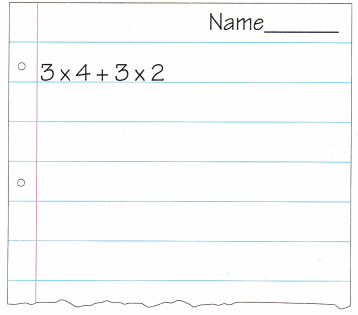So, ________ cups of flour and sugar are needed.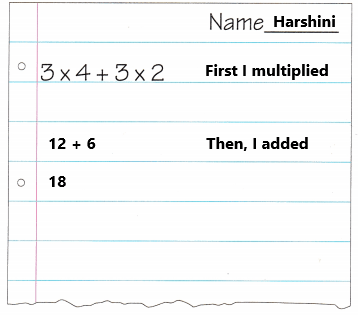So, 18 cups of flour and sugar are needed.
Explanation:
A cake recipe calls for 4 cups of flour and 2 cups of sugar. The recipe was tripled. The expression is 3 x 4 + 3 x 2. So first I multiplied 3 with 4 and 3 with 2 the product is 12 and 6. Then I added 12 with 6 the sum is 18.

Example

Each batch of cupcakes Lena makes uses 3 cups of flour, 1 cup of milk, and 2 cups of sugar. Lena wants to make 5 batches of cupcakes. How many cups of flour, milk, and sugar will she need in all?
Write the expression. 5 × (3 + 1 + 2)
I First, perform the operations in parentheses. 5 × (_________)
Then multiply. _________
So, Lena will use _________ cups of flour, milk, and sugar in all.
The expression is 5 x (3 + 1 + 2).
I First, perform the operations in parentheses.
5 × (3 + 1 + 2)
5 x (6)
Then multiply.
5 x 6
30
So, Lena will use 30 cups of flour, milk, and sugar in all.
Explanation:
Each batch of cupcakes Lena makes uses 3 cups of flour, 1 cup of milk, and 2 cups of sugar. Lena wants to make 5 batches of cupcakes. The expression is 5 × (3 + 1 + 2). First perform the operations in parentheses. Add 3 with 1 and 2 the sum is 6. Then multiply 5 with 6 the product is 30. So, Lena will use 30 cups of flour, milk, and sugar in all.

Share and Show

Simplify the numerical expression.

Question 1.
10 + 36 ÷ 9
Think: I need to divide first.
10 + 36 ÷ 9
10 + 4
14
Explanation:
The numerical expression is 10 + 36 ÷ 9. First I divided 36 by 9 the result is 4. Then I added 10 with 4 the sum is 14. The simplified form of given numerical expression is 14.

Question 2.
10 + (25 – 10) ÷ 5
10 + (25 – 10) ÷ 5
10 + 15 ÷ 5
10 + 3
13
Explanation:
The numerical expression is 10 + (25 – 10) ÷ 5. First I Subtracted 10 from 25 then the difference is 15. Next I divided 15 by 5 the result is 3. Then I added 10 with 3 the sum is 13. The simplified form of given numerical expression is 13.

Question 3.
9 – (3 × 2) + 8
9 – (3 × 2) + 8
9 – (6) + 8
3 + 8
11
Explanation:
The numerical expression is 9 – (3 × 2) + 8. First I multiplied 3 with 2 the product is 6. Next I subtracted 6 from 9 the difference is 3. Then I added 3 with 8 the sum is 11.The simplified form of given numerical expression is 11.

Math Talk
Mathematical Processes

Raina simplified the expression 5 × 2 + 2 by adding first and then multiplying. Will her answer be correct? Explain.
5 × 2 + 2
5 x 4
20
No, her answer is 20 it is not correct. The correct answer is 12.
Explanation:
Raina simplified the expression 5 × 2 + 2 by adding first and then multiplying. Her answer will not be correct. Because Raina has to perform multiplication first and then adding.

Problem Solving

Question 4.
H.O.T. Use Symbols Write 12 + 17 – 3 × 2 with parentheses so it has a value of 23.
One type:
12 + 17 – (3 x 2)
12 + 17 – 6
29 – 6
23
Another type:
(12 + 17) – 3 x 2
29 – 6
23
Explanation:
The parentheses for the expression 12 + 17 – 3 × 2 is 12 + 17 – (3 x 2) or (12 + 17) – 3 x 2. By solving these numerical expression with parentheses the value is 23.

Question 5.
Multi-Step The value of 100 – 30 ÷ 5 with parentheses can have a value of 14 or 94. Explain.
One type:
100 – (30 ÷ 5)
100 – 6
94
The value of the given expression with the above parentheses is 94.
100 – (30 ÷ 5)
70 ÷ 5
14
The value of the given expression with the above parentheses is 14.
Explanation:
The two possible ways of the given expression with parentheses is 100 – (30 ÷ 5), 100 – (30 ÷ 5). By solving these two expression the values is 94 and 14.

Unlock the Problem

Question 6.
Multi-Step A movie theater has 4 groups of seats. The largest group of seats, in the middle, has 20 rows, with 20 seats in each row. There are 2 smaller groups of seats on the sides, each with 20 rows and 6 seats in each row. A group of seats in the back has 5 rows, with 30 seats in each row. How many seats are in the movie theater?a. What do you need to know?
We need to know the total seats in the movie theater.

b. What operation can you use to find the number of seats in the back group of seats? Write the expression.
The expression is 5 x 30.
150
Explanation:
Multiplication operation is used to find the number of seats in the back group of seats. A group of seats in the back has 5 rows, with 30 seats in each row. The expression is 5 x 30. There are 150 seats in the back group of seats.

c. What operation can you use to find the number of seats in both groups of side seats? Write the expression.
The expression is 2 x (20 x 6).
2 x 120
240
Explanation:
Multiplication operation is used to find the number of seats in both groups of side seats. There are 2 smaller groups of seats on the sides, each with 20 rows and 6 seats in each row. The expression is 2 x (20 x 6). There are 240 seats in both groups of side seats.

d. What operation can you use to find the number of seats in the middle group? Write the expression.
The expression is 20 x 20.
400
Explanation:
Multiplication operation is used to find the number of seats in the middle group. The largest group of seats, in the middle, has 20 rows, with 20 seats in each row. The expression is 20 x 20. There are 400 seats in the middle group.

e. Write an expression to represent the total number of seats in the theater.
The expression to represent the total number of seats in the theater is (20 x 20) + 2 x (20 x 6) + (5 x 30).

f. How many seats are in the theater? Show the steps you use to solve the problem.
(20 x 20) + 2 x (20 x 6) + (5 x 30)
400 + 2 x 120 + 150
400 + 240 + 150
790
The total number of seats in the theater is 790.

Question 7.
Multi-Step In the wild, an adult giant panda eats about 30 pounds of food each day. Write and simplify a numerical expression that shows how many pounds of food 6 pandas eat in 3 days.
3 x (6 x 30)
3 x 180
540
In 3 days 6 pandas eat 540 pounds of food.
Explanation:
In the wild, an adult giant panda eats about 30 pounds of food each day. The numerical expression for the 6 pandas eats food in 3 days is 3 x (6 x 30). In 3 days 6 pandas eat 540 pounds of food.

Question 8.
H.O.T. Connect Write and simplify two equivalent numerical expressions that show the Distributive Property of Multiplication.Question 9.
The books in an unusual library are labeled with numerical expressions. Katrina needs to find the book with a numerical expression that has a value of 12. Which one should she choose?
(A) (6 + 26) – (4 × 5)
(B) 2 × (15 – 7)
(C) 10 + 8 – 36 ÷ 9
(D) (25 – 4) ÷ 7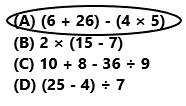(6 + 26) – (4 × 5)
32 – 20
12
The book with a numerical expression that has a value of 12 is (6 + 26) – (4 × 5).
So, option A is correct.
Explanation:
The books in an unusual library are labeled with numerical expressions. Katrina needs to find the book with a numerical expression that has a value of 12. The book with a numerical expression is (6 + 26) – (4 × 5). First we have to add 6 with 26 the sum is 32. Next multiply 4 with 5 the result is 20. Then subtracting 20 from 32 the difference is 12. So, option A is correct.

Question 10.
Franco needs to simplify 3 × (8 – 4) + 7. What should be his first step?
(B) Subtract 4 from 8.
(C) Multiply 3 by 7.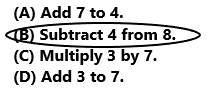3 × (8 – 4) + 7
3 x 4 + 7
12 + 7
19
The first step is to solve (8 – 4).
So, option B is correct.
Explanation:
The given numerical expression is 3 × (8 – 4) + 7. First we have to solve the values within the parentheses.  Subtract 4 from 8 the difference is 4. Next we have to perform multiplication operation. Multiply 3 with 4 the result is 12. Then add 12 with 7 the sum is 19.

Question 11.
Multi-Step Sarah simplified each of the expressions shown. What is the difference between the values of the two expressions?
3 × 12 – 9 + 10
3 × (12 – 9) + 10
(A) 9
(B) 18
(C) 12
(D) 223 × 12 – 9 + 10
36 – 9 + 10
27 + 10
37
3 × (12 – 9) + 10
3 x 3 + 10
9 + 10
19
37 – 19 = 18
The difference between the values of the two expression is 18.
So, option B is correct.
Explanation:
Sarah simplified each of the given expressions. The first expression is 3 × 12 – 9 + 10. First Sarah multiplied 3 with 12 the product is 36. Then she subtracted 9 from 36 the difference is 27. Then she added 27 with 10 the sum is 37.  The second expression is 3 × (12 – 9) + 10. First Sarah subtracted 9 from 12 the difference is 3. Then she multiplied 3 with 3 the product is 9. The she added the 9 with 10 the sum is 18. Subtract 18 from 37 the difference is 18. The difference between the values of the two expression is 18. So, option B is correct.

Texas Test Prep

Question 12.
Which expression has a value of 6?
(A) (6 ÷ 3) × 4 + 8
(B) 27 – 9 ÷ 3 × (4 + 1)
(C) (18 + 12) × 6 – 4
(D) 71 – 5 × (9 + 4)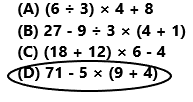71 – 5 × (9 + 4)
71 – 5 x (13)
71 – 65
6
The expression that has a value of 6 is 71 – 5 × (9 + 4).
So, option D is correct.
Explanation:
The expression that has a value of 6 is 71 – 5 × (9 + 4). First add the values within the parentheses. Add 9 with 4 the sum is 13. Then multiply 5 with 13 the product is 65. Then subtract 65 from 71 the difference is 6.

### Texas Go Math Grade 5 Lesson 7.4 Homework and Practice Answer Key

Simplify the numerical expression.

Question 1.
10 – (2 × 4) + 12
The given expression is 10 – (2 × 4) + 12.
10 – 8 + 12
2 + 12
14
Explanation:
The given expression is 10 – (2 × 4) + 12. First simplify the values within the parentheses. Multiply 2 with 4 the product is 8. Then subtract 8 from 10 then the difference is 2. Then add 2 with 12 the sum is 14. The simplified form of given numerical expression is 14.

Question 2.
14 + 35 ÷ 7
The given expression is 14 + 35 ÷ 7.
14 + 5
19
Explanation:
The given expression is 14 + 35 ÷ 7. First divide 35 by 7 the result is 5. Then add 5 with 14 the sum is 19. The simplified form of given numerical expression is 19.

Question 3.
11 + (31 – 4) ÷ 9
The given expression is 11 + (31 – 4) ÷ 9.
11 + 27 ÷ 9
11 + 3
14
Explanation:
The given expression is 11 + (31 – 4) ÷ 9. First simplify the values within the parentheses. Subtract 4 from 31 the difference is 27. Then divide 27 by 9 the result is 3. Then add 11 with 3 the sum is 14. The simplified form of given numerical expression is 14.

Question 4.
25 – 4 × 5
The given expression is 25 – 4 × 5.
25 – 20
5
Explanation:
The given expression is 25 – 4 × 5. First multiply 4 with 5 the product is 20. Then subtract 20 from 25 the difference is 5. The simplified form of given numerical expression is 5.

Question 5.
3 × (6 + 3) – 15
The given expression is 3 × (6 + 3) – 15.
3 x 9 – 15
27 – 15
12
Explanation:
The given expression is 3 × (6 + 3) – 15. First simplify the values within the parentheses. Add 6 with 3 the sum is 9. Then multiply 3 with 9 the product is 27. Subtract 15 from 27 the difference is 12. The simplified form of given numerical expression is 12.

Question 6.
30 – 14 ÷ 2
The given expression is 30 – 14 ÷ 2.
30 – 7
23
Explanation:
The given expression is 30 – 14 ÷ 2. First divide 14 by 2 the result is 7. Then subtract 7 from 30 the difference is 23. The simplified form of given numerical expression is 23.

Rewrite the expression with parentheses to equal the given value.

Question 7.
12 + 6 ÷ 2 + 4; value: 13
The given expression is 12 + 6 ÷ 2 + 4.
Rewrite the expression with parentheses.
12 + 6 ÷ (2 + 4)
12 + 6 ÷ 6
12 + 1
13
Explanation:
The given expression 12 + 6 ÷ 2 + 4 had rewrite with parentheses 12 + 6 ÷ (2 + 4) to equal to the given value. The given expression with parentheses is equal to the given value 17.

Question 8.
42 – 24 ÷ 6; value: 3
The given expression is 42 – 24 ÷ 6.
Rewrite the expression with parentheses.
(42 – 24) ÷ 6
18 ÷ 6
3
Explanation:
The given expression 42 – 24 ÷ 6 had rewrite with parentheses (42 – 24 )÷ 6 to equal to the given value. The given expression with parentheses is equal to the given value 17.

Question 9.
9 + 16 – 2 × 4; value: 17
The given expression is 9 + 16 – 2 × 4.
Rewrite the expression with parentheses.
9 + 16 – (2 × 4)
9 + 16 – 8
9 + 8
17
Explanation:
The given expression 9 + 16 – 2 × 4 had rewrite with parentheses  9 + 16 – (2 × 4) to equal to the given value. The given expression with parentheses is equal to the given value 17.

Question 10.
60 – 3 + 2 × 5; value: 35
The given expression is 60 – 3 + 2 × 5.
Rewrite the expression with parentheses.
60 – (3 + 2) × 5
60 – 5 x 5
60 – 25
35
Explanation:
The given expression 60 – 3 + 2 × 5 had rewrite with parentheses  60 – (3 + 2) × 5 to equal to the given value. The given expression with parentheses is equal to the given value 35.

Question 11.
18 + 9 ÷ 3; value: 21
The given expression is 18 + 9 ÷ 3.
Rewrite the expression with parentheses.
18 + (9 ÷ 3)
18 + 3
21
Explanation:
The given expression 18 + 9 ÷ 3 had rewrite with parentheses 18 + (9 ÷ 3) to equal to the given value. The given expression with parentheses is equal to the given value 21.

Question 12.
5 × 2 + 4 + 3; value: 45
The given expression is 5 × 2 + 4 + 3.
Rewrite the expression with parentheses.
5 × (2 + 4 + 3)
5 x 9
45
Explanation:
The given expression 5 × 2 + 4 + 3 had rewrite with parentheses 5 × (2 + 4 + 3) to equal to the given value. The given expression with parentheses is equal to the given value 45.

Problem Solving

Question 13.
Julian paid his library fines. He paid $2 each for 4 overdue books and$16 for a lost book. Write and simplify a numerical expression that shows how much Julian paid in library fines.
The numerical expression is as below.
$2 x 4 +$16
$8 +$16
$24 Julian paid$24 in library fines.
Explanation:
Julian paid his library fines. He paid $2 each for 4 overdue books and$16 for a lost book. The numerical expression is $2 x 4 +$16. The simplified form of numerical expression value is $24. Julian paid$24 in library fines.

Question 14.
For 5 days in a row last week, Addison read 9 pages of a poetry book and 16 pages of her favorite novel. Write and simplify a numerical expression that shows how many pages Addison read last week.
5 x (9 + 16)
5 x 25
125
Explanation:
For 5 days in a row last week, Addison read 9 pages of a poetry book and 16 pages of her favorite novel. The numerical expression is 5 x (9 + 16). The simplified form of numerical expression value is 125. Addison read 125 pages last week.

Lesson Check

Question 15.
Mrs. Harrison writes a numerical expression on the board that has a value of 16. Which expression does Mrs. Harrison write?
(A) (15 + 3) – 14 ÷ 2
(B) 20 – 4 ÷ 2
(C) 3 × (5 + 1)
(D) 18 + 13 – (5 × 3)18 + 13 – (5 × 3)
18 + 13 – 15
31 – 15
16
So, option D is correct.
Explanation:
Mrs. Harrison writes a numerical expression on the board that has a value of 16. Mrs. Harrison wrote 18 + 13 – (5 × 3) expression on the board. The value of this expression is equal to 16.

Question 16.
Omar wants to simplify the expression below. What should be his first step?
6 × (3 + 9) – 5
(A) Multiply 6 by 3.
(B) Subtract 5 from 9.
(D) Subtract 5 from 6.6 × (3 + 9) – 5
6 x 12 – 5
72 – 5
67
Omar first step is to add 9 to the 3.
Explanation:
Omar wants to simplify the expression 6 × (3 + 9) – 5. First Omar has to solve the values within the parentheses. So, Omar’s first step is to add 9 to 3. So, option C is correct.

Question 17.
Which is the value of the numerical expression below?
30 ÷ (3 + 2) × 8
(A) 96
(B) 48
(C) 26
(D) 40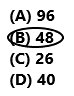30 ÷ (3 + 2) × 8
30 ÷ 5 x 8
6 x 8
48
So, option B is correct.
Explanation:
The given numerical expression is 30 ÷ (3 + 2) × 8. First add 3 with 2 the sum is 5. Then divide 30 by 5 the result is 6. Then after multiply 6 with 8 the product is 48. So, option B is correct.

Question 18.
Becka says that when she simplifies the expression below, the value is 6 less than her age. What is Becka’s age?
(7 + 11) – 8 ÷ 2
(A) 20
(B) 14
(C) 26
(D) 11(7 + 11) – 8 ÷ 2
18 – 8 ÷ 2
18 – 4
14
20 – 6 = 14
Becka’s age is 20.
So, option A is correct.
Explanation:
Becka said that when she simplifies the given expression (7 + 11) – 8 ÷ 2, the value is 6 less than her age. The simplified form of the given expression is 14. Becka’s age is 20. So, option A is correct.

Question 19.
Multi-Step Kalani and Porter each simplified one of the expressions below. If they add their results together, what will be their sum?
Kalani: 4 × 11 -5 + 12
Porter: 4 × 11 – (5 + 12)
(A) 78
(B) 44
(C) 102
(D) 54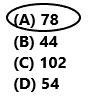Kalani: 4 × 11 -5 + 12
44 – 5 + 12
39 + 12
51
Porter: 4 × 11 – (5 + 12)
44 – 17
27
51 + 27 = 78
So, option A is correct.
Explanation:
Kalani simplified the given expression 4 × 11 -5 + 12. The value of the Kalani’s expression is 51. Porter simplified the given expression 4 × 11 – (5 + 12). The value of the Porter expression is 27. They added their results together. Add 51 with 27 the sum is 78. So, option A is correct.

Question 20.
Multi-Step Nicole simplified the expression below. Then she multiplied the value of the expression by 5. What is Nicole’s product?
36 + 6 ÷ 3 + 2
(A) 16
(B) 80
(C) 40
(D) 200